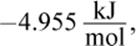﻿ ﻿Answers and Explanations - Thermochemistry - Training MCAT General Chemistry Review

## MCAT General Chemistry Review

### Chapter 7: Thermochemistry

1. AThis process may be adiabatic. Given that the gas was cooled, it did not maintain constant temperature, eliminating choice (C). Isobaric and isovolumetric processes appear as horizontal and vertical lines in pressure–volume graphs, respectively, eliminating choices (B) and (D). Adiabatic processes appear hyperbolic on pressure–volume graphs, as illustrated here.

2. DThere is not enough information in the problem to determine whether or not the reaction is spontaneous. If the signs of enthalpy and entropy are the same, the reaction is temperature dependent according to ΔG = ΔHTΔS. Without the temperature, we cannot determine if this reaction is spontaneous, nonspontaneous, or at equilibrium.

3. DSolve this question using the equation ΔG°rxn = −RT ln Keq. ΔGrxn° is negative (as it must be for a spontaneous reaction), and R and T are always positive. Therefore, ln Keq must also be positive for the sign convention to work out correctly. If ln Keq > 0, then eln Keq > e0Keq > 1.

4. DCombustion often involves the reaction of a hydrocarbon with oxygen to produce carbon dioxide and water. Longer hydrocarbon chains yield greater amounts of combustion products and release more heat in the process—that is, the reaction is more exothermic. Of the hydrocarbons listed here, n-pentane is the longest chain.

5. AAt first glance, this might seem like a math-heavy problem, but it really doesn’t require any calculations at all. We just have to keep track of which bonds are broken and which bonds are formed. Remember, breaking bonds requires energy, while forming bonds releases energy. Two bonds are broken: a C–O bond between the carbon and oxygen of methanol, and an O–H bond between the hydroxyl oxygen and hydrogen of the acetic acid. Two bonds are also formed: a C–O bond between the esterifying group and the oxygen of the methyl acetate, and an O–H bond between the hydroxyl group and a hydrogen to form water. Given that the same two bonds are broken and formed in this reaction, the energy change must be6. CStandard temperature and pressure indicates 0°C and 1 atm. Gibbs free energy is temperature dependent, but if a reaction is at equilibrium, ΔG = 0.

7. B

Solve this question using the equation ΔG°rxn = −RT ln Keq. ΔGrxn° isR isand T = 298 K because the reaction is occurring under standard conditions. Because ΔG°rxn uses kilojoules in its units and R uses joules, one will have to be converted. Plugging into the equation, we get:If ln Keq = 2, then Keq = e2. e is approximately 2.7, so we’re looking for a number between 4 (22) and 9 (32). Both choices (B) and (C) fit these criteria; however, 8.9 is very close to 9, so we can assume that its square root is very, very close to 3. The answer choice should be a bit smaller, so choice (B), 7.4, is correct.

8. DThere is not enough information available to determine the free energy of this reaction. While the entropy is clearly increasing (there are more particles in the system), it is unclear what the enthalpy change is. Because bonds are breaking, the reaction must be endothermic, meaning that both ΔS and ΔH are positive. In this case, it is a temperature-dependent process, and—without a temperature given—we cannot determine the sign on ΔG.

9. AThis problem asks for the free energy of a reaction at nonstandard conditions, which can be determined with the equation ΔG = ΔG° + RT ln Q.

10.AA reaction with a negative enthalpy is, by definition, exothermic. Because both enthalpy and entropy are negative, this is a temperature-dependent process, and the reaction will be both endergonic and exergonic—but only at particular temperatures, eliminating choices (C) and (D).

11.CFor a process to progress forward spontaneously, Q must be less than Keq and will therefore have a tendency to move in the direction toward equilibrium. A spontaneous reaction’s free energy is negative by convention.

12.BA calorimeter measures heat transfer. Although calorimeters often incorporate thermometers, the thermometer itself only tracks temperature, not the heat transfer itself, so choice (A) is incorrect. Choice (C) is irrelevant; barometers measure changes in pressure. Choice (D) is also incorrect because volumetric flasks measure quantities of liquid, not the heat capacity of the liquid.

13.AEliminate choices (C) and (D), which describe the free energy of reaction and cannot be determined from this graph. While most reaction coordinate graphs we’ve explored in this book use free energy for the y-axis, this one uses potential energy (enthalpy). If the heat of formation of the products is greater than that of the reactants, the reaction is endothermic. We can determine this information from their relative positions on the graph: because the products are higher than the reactants, this is an endothermic reaction.

14.CGases have the highest entropy, and solids have the lowest. Therefore, a phase change from a gas to a solid—deposition—would have the largest decrease in entropy of any phase change process.

15.AIn an explosion, a significant amount of heat energy is released, meaning that the reaction is exothermic (ΔH < 0), eliminating choice (B). The entropy change associated with an explosion is positive because energy is dispersed over a much larger area, eliminating choice (C). If this is true, the expression ΔHTΔS must be negative, indicating that this is an exergonic process (ΔG < 0). Absolute temperature can never be negative, eliminating choice (D).

﻿